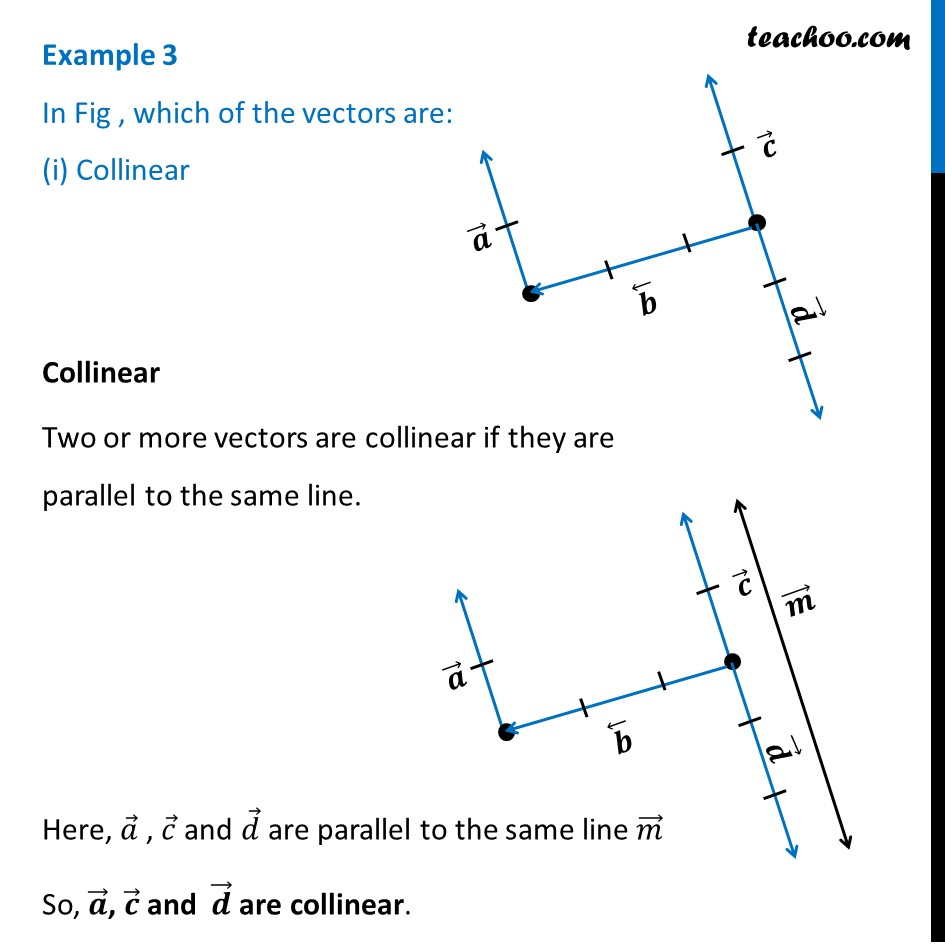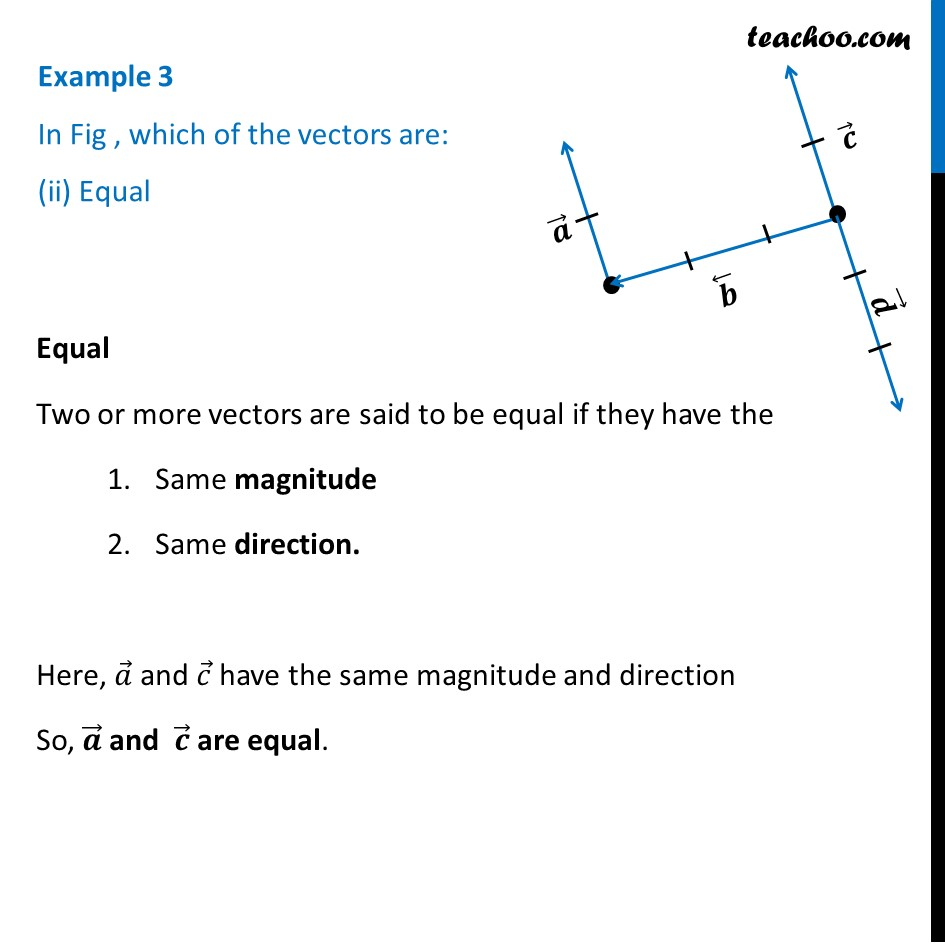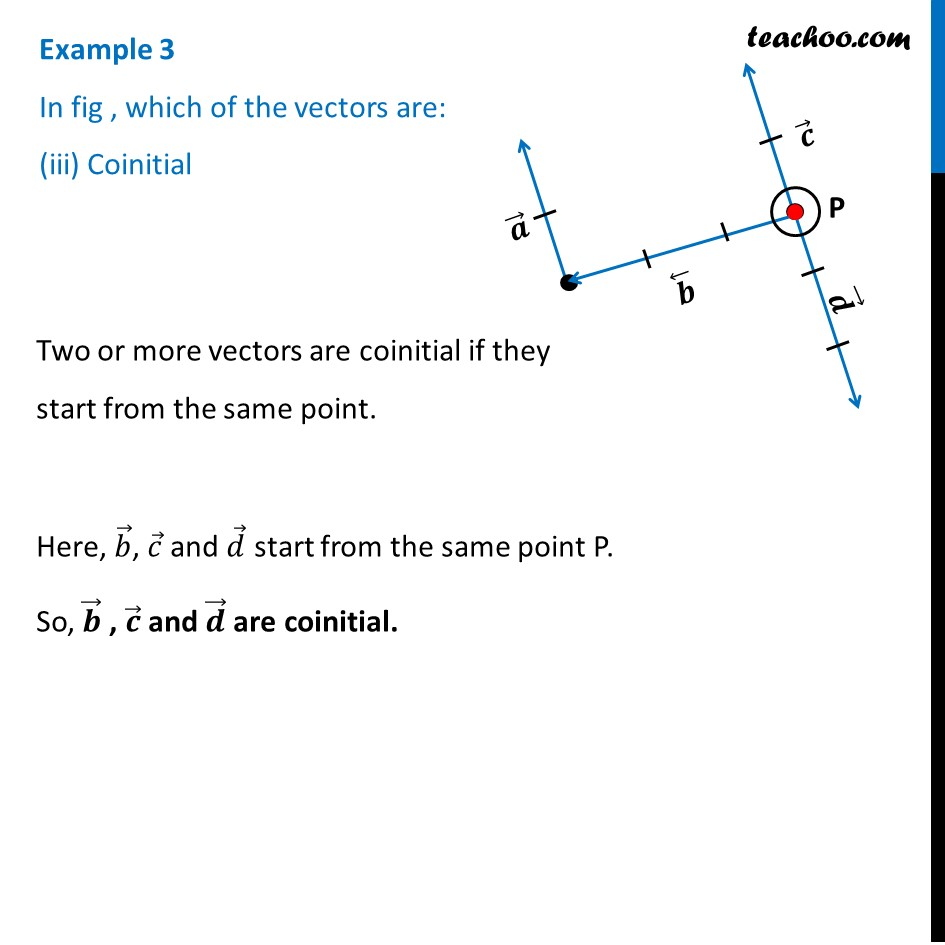Examples

Chapter 10 Class 12 Vector Algebra
Serial order wiseLearn in your speed, with individual attention - Teachoo Maths 1-on-1 Class

### Transcript

Example 3 In Fig , which of the vectors are: (i) CollinearCollinear Two or more vectors are collinear if they are parallel to the same line. Here, 𝑎 ⃗ , 𝑐 ⃗ and 𝑑 ⃗ are parallel to the same line 𝑚 ⃗ So, 𝒂 ⃗, 𝒄 ⃗ and 𝒅 ⃗ are collinear. Example 3 In Fig , which of the vectors are: (ii) Equal Equal Two or more vectors are said to be equal if they have the Same magnitude Same direction. Here, 𝑎 ⃗ and 𝑐 ⃗ have the same magnitude and direction So, 𝒂 ⃗ and 𝒄 ⃗ are equal. Example 3 In fig , which of the vectors are: (iii) Coinitial Two or more vectors are coinitial if they start from the same point. Here, 𝑏 ⃗, 𝑐 ⃗ and 𝑑 ⃗ start from the same point P. So, 𝒃 ⃗ , 𝒄 ⃗ and 𝒅 ⃗ are coinitial.